# Chapter 5.2. Loops – Exam Problems

In the previous chapter, we learned how to execute a block of commands more than once. That’s why we introduced for loops and we looked at some of its main use cases. This chapter aims to consolidate our knowledge by solving some more complex problems with loops, given at exams. We will show detailed solutions for some of the examples, and for the others, we will leave guidance only. Before we get to work it’s best if we recall the construction of the for loop: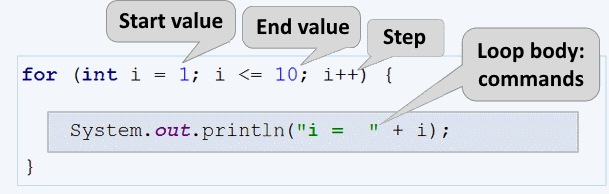For loops consist of:

• Initialization block - executed only once at the beginning before the compiler checks the repeat condition for the first time. In this case, the variable counter (int i) is declared and its initial value is set.
• Condition for repeatability (i <= 10), ran once before each iteration.
• Counter update (i++) – this code is executed after each iteration.
• Loop body - contains an arbitrary block of source code.

## Exam Problems

Let’s solve some loop problems from exams in SoftUni.

### Problem: Histogram

An n number of integer numbers are given in the range [1 … 1000]. Some percent of them p1 are below 200, percent p2 are from 200 to 399, percent p3 are from 400 to 599, percent p4 are from 600 to 799 and the rest are p5 percent above 800 (inclusive). Write a program that calculates and prints the percentages p1, p2, p3, p4, and p5 on the console.

Example: we have n = 20 and the numbers: 53, 7, 56, 180, 450, 920, 12, 7, 150, 250, 680, 2, 600, 200, 800, 799, 199, 46, 128, 65. We get the following distribution and visualization:

Group Numbers Number count Percent
< 200 53, 7, 56, 180, 12, 7, 150, 2, 199, 46, 128, 65 12 p1 = 12 / 20 * 100 = 60.00%
200 … 399 250, 200 2 p2 = 2 / 20 * 100 = 10.00%
400 … 599 450 1 p3 = 1 / 20 * 100 = 5.00%
600 … 799 680, 600, 799 3 p4 = 3 / 20 * 100 = 15.00%
≥ 800 920, 800 2 p5 = 2 / 20 * 100 = 10.00%

### Input Data

In the first line of the input, there must be an integer number n (1 ≤ n ≤ 1000), which represents how many lines of numbers we should enter. In each of the following n lines, we have one integer number in the range [1 … 1000] – those are the numbers we use to calculate the histogram.

### Output Data

Print on the console a histogram of 5 lines, each line should contain a percentage from 0% to 100%, and format it up to two digits after the decimal point (for example 25.00%, 66.67%, 57.14%).

### Sample Input and Output

Input Output Input Output
3
1
2
999
66.67%
0.00%
0.00%
0.00%
33.33%
4
53
7
56
999
75.00%
0.00%
0.00%
0.00%
25.00%
Input Output Input Output
7
800
801
250
199
399
599
799
14.29%
28.57%
14.29%
14.29%
28.57%
9
367
99
200
799
999
333
555
111
9
33.33%
33.33%
11.11%
11.11%
11.11%
Input Output
14
53
7
56
180
450
920
12
7
150
250
680
2
600
200
57.14%
14.29%
7.14%
14.29%
7.14%

### Hints and Guidelines

The program that solves this problem can be imaginatively split into three parts:

• Reading the input data – in the current problem this involves reading the number n, followed by n count of integers, each on a separate line.
• Processing the input data – in this case, this means allocating the numbers into groups and calculating the percentage split by a group.
• Printing the final result – printing the histogram to the console in the specified format.

Before we continue, we will take a small deviation from the current topic, namely, we will briefly mention, that in coding every variable is of some kind of data type. We will use numeric data types int for integer numbers and double for real numbers in this problem.

Now we are going to proceed with the implementation of the above-made points.

Before we move on to reading the input data we have to declare the variables, in which we are storing them. This means that we have to choose the right data type and appropriate names.In the variable n, we will store the count of numbers, that we are going to read from the console. We choose int type, because the condition states, that n is an integer number in the range from 1 to 1000. For the variables in which we will store the percentages, we chose the double type, because they are not always expected to be integer numbers. Additionally, we declare the variables cntP1, cntP2, etc, in which we will store the count of numbers in the corresponding group, and for them, we choose the int type again.

Once we have declared the needed variables, we can proceed with reading the number n from the console: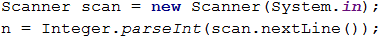#### Processing The Input Data

To read and distribute each number to its corresponding group, we will use a for loop from 0 to n (count of numbers). Each iteration of the loop will read and distribute only one number (currentNumber) to its corresponding group. For the compiler to determine if a number belongs to a group, we perform a check-in its range. If so- we add to the count of its corresponding group (cntP1, cntP2, etc.) by one.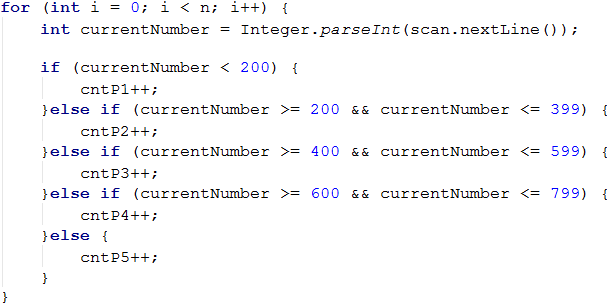After we determined how many numbers each group has, we can move on to calculating the percentage, which is the main point of the problem. For this, we’ll use the following formula:

(group percentage) = (count of numbers in the group) * 100 / (count of all numbers)

This formula is the program code looks like the following: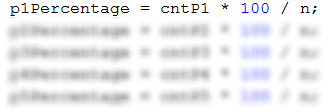If we divide by 100 (int number type) instead of 100.0 (double number type), the so-called integer division will be performed and only the whole part of the division will be stored in the variable, which is not the desired result. For example: 5 / 2 = 2, but 5 / 2.0 = 2.5. Taking this in mind, the formula for the first number should look like this: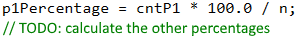To make things even clearer, let’s take a look at this example:

Input Output
3
1
2
999
66.67%
0.00%
0.00%
0.00%
33.33%

In the case of n = 3. For the loop we have:

• i = 0 - we read the number 1, which is less than 200 and falls in the first group (p1), and we increment the group counter (cntP1) by 1.
• i = 1 – we read the number 2, which again falls in the first group (p1) and we increment the counter (cntP1) again by 1.
• i = 2 – we read the number 999, which falls in the last group (p5), because it is greater than 800, and we increment the group counter (cntP5) by 1.

After reading the numbers in group p1 we have 2 numbers, and in p5 we have 1 number. We have no numbers in the other groups. Applying the above formula, we calculate the percentages of each group. If we multiply within the formula by 100, instead of 100.0 we’ll attain 66% for group p1, and 33% for group p5 (there will be no fractional part).

#### Final Output

All that remains is to print the results. The condition states, that the percentages must be accurate to two digits after the decimal point. We can achieve this by setting the format to “%.2f%%” in the String.format(…) method: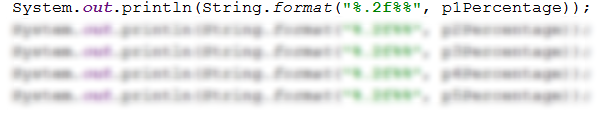### Problem: Smart Lilly

Lilly is N years old. For every birthday she receives a present. For each odd birthday (1, 3, 5, …, n), she receives toys, and for every even birthday (2, 4, 6, …, n), she receives money. For her second birthday, she received 10.00 USD., and the number of money increases by 10.00 USD for each following even birthday (2 -> 10, 4 -> 20, 6 -> 30, etc.). Over the years Lilly secretly saved her money. Her brother, in the years when Lilly received money, takes 1.00 USD of it. Lilly has sold the toys, received over the years, each for P USD, and added the money to her savings. With the money, she wanted to buy a washing machine for X USD. Write a program that calculates how much money she has saved and if the amount is enough to buy a washing machine.

### Input Data

The console reads 3 numbers, each on a separate line:

• Lilly’s age – an integer number in the range of [1 … 77].
• Price of the washing machine – a double number in the range of [1.00 … 10 000.00].
• Unit price per toy – an integer number in the range of [0 … 40].

### Output Data

Print on the console a single line:

• If Lilly’s money is enough:
• Yes! {N}” – where N is the remaining money after the purchase.
• If the money is not enough:
• No! {M}” – where M is the insufficient amount.
• The numbers N and M must be formatted up to the second digit after the decimal point.

### Sample Input and Output

10
170.00
6
Yes! 5.00

For her first birthday, she receives a toy; 2nd -> 10 USD; 3rd -> toy; 4th -> 10 + 10 = 20 USD; 5th -> toy; 6th -> 20 + 10 = 30 USD; 7th -> toy; 8th -> 30 + 10 = 40 USD; 9th -> toy; 10th -> 40 + 10 = 50 USD.
She has saved -> 10 + 20 + 30 + 40 + 50 = 150 USD. She sold 5 toys for 6 USD each = 30 USD..
Her brother took 1 USD 5 times = 5 USD. Remaining amount -> 150 + 30 – 5 = 175 USD. 175 >= 170 (price of the washing machine) She was able to buy it and has a remaining amount of money 175-170 = 5 USD.

21
1570.98
3
No! 997.98

She has saved 550 USD. She sold 11 toys 3 USD each = 33 USD. Her brother has taken for 10 years 1 USD each year = 10USD. The remainder 550 + 33 – 10 = 573 USD.
573 < 1570.98she did not manage to buy a washing machine. The insufficiency amount is 1570.98–573 = 997.98 USD.

### Hints and Guidelines

The solution to this problem, like the previous one can be split into three parts – reading the input data, processing it, and printing the output.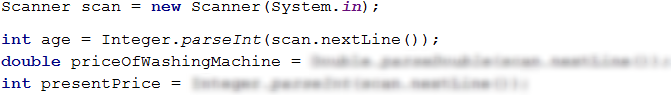We begin again by choosing the appropriate data types and names of the variables. Lilly’s years (age) and the toy's unit price (presentPrice) are conditionally given to be whole numbers. That’s why we’ll use the int type. For the washing machine price (priceOfWashingMachine) we know, that it is a real number and we choose double. In the code above we declare and initialize (assign a value to) the variables.To solve the problem, we will need several auxiliary variables – for the toy count (numberOfToys), for the money saved (savedMoney), and for the money she received each birthday (moneyForBirthday). The initial value of moneyForBirthday is 10 because the description states the first sum Lilly got is 10 USD.Using a for loop we iterate through each of Lilly’s birthdays. When the counter in our loop (which equates to Lilly’s age) is an even number, this means that Lilly receives money and we add this money to her total savings accordingly. Simultaneously we subtract 1 USD - the money her brother takes. Then we increase the variable value moneyForBirthday, i.e. we increase by 10 the sum she will get on her next birthday. The opposite of that, when the counter (Lilly's age) is an odd number, we increase the toy counter. We do the parity check by division with a remainder (%) by 2 – when the remainder is 0, the number is even, when we have a remainder of 1 - it is odd.

We also add the money from the sold toys to Lilly’s savings: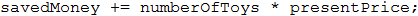Finally, we need to print the results, following the formatting specified in the condition, i.e. the sum must be rounded up to 2 digits after the decimal point: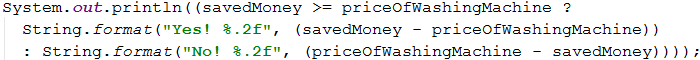In this case, we chose to use the conditional operator (?:) (also called a ternary operator), because it’s shorter to write. The syntax is as follows: operand1 ? operand2 : operand3. The first operand needs to be boolean type (i.e. to return true/false). If operand1 returns true, operand2 will be executed, and if it returns falseoperand3 will be executed. In our case, we check if the saved money by Lilly is enough for the washing machine. If they are more or equal to the price of the washing machine, the check savedMoney >= priceOfWashingMachine will return true and will print out „Yes! …“, and if they are less the result will be false and will print “No! …”. Of course, instead of the ternary operand, we can use if-else checks.

More about the ternary operator: https://www.programiz.com/java-programming/ternary-operator

### Problem: Back to The Past

Ivan is 18 years old and receives an inheritance, that consists of X sum of money and a time machine. He decides to go back to the year 1800, but he doesn’t know if the money is enough to live without working. Write a program that calculates if Ivan is going to have enough money, so he won’t need to work until a particular year (inclusive). Assuming that for every even (1800, 1802, etc.) year he will spend 12 000 dollars. For every odd (1801,1803 etc.) year he will spend 12 000 + 50 * [the age he reached in the given year].

### Input Data

The input is read from the console and contains exactly 2 lines:

• Inheritance money – a real number in the range of [1.00 … 1 000 000.00].
• The year he needs to live to (inclusive) – a whole number in the range of [1801 … 1900].

### Output Data

Print on the console 1 line. The sum must be formatted up to two symbols after the decimal point:

• If the money is enough:
• Yes! He will live a carefree life and will have {N} dollars left.“ – where N is the money, which he will remain with.
• If the money is NOT enough:
• He will need {M} dollars to survive.“ – where M is the amount that is NOT enough.

### Sample Input and Output

Input Output Explanations
50000
1802
Yes! He will live a carefree life and
will have 13050.00 dollars left.

1800 → even
→ Spends 12000 dollars
→ Remain 50000 – 12000 = 38000
1801 → odd
→ Spends 12000 + 19*50 = 12950 dollars
→ Remaining 38000 – 12950 = 25050
1802 → even
→ Spends 12000 dollars
→ Remaining 25050 – 12000 = 13050

100000.15
1808
He will need 12399.85 dollars
to survive.

1800 → even
→ Remaining 100000.15 – 12000 = 88000.15
1801 → odd
→ Remaining 88000.15 – 12950 = 75050.15

1808 → even → -399.85 - 12000 = -12399.85
12399.85 insufficient amount

### Hints and Guidelines

The method for solving this problem isn’t much different from the last one, we begin by declaring and initializing the needed variables:The condition states, that Ivan is 18 years old, so when we declare the variable years we assign its initial value of 18. The rest of the variables are read from the console.With the help from the for loop, we’ll iterate through all the years. We begin with the year 1800 – the year that Ivan goes back to, and we get to the year he has to live to. In the loop, we check if the current year is even or odd. We achieve this by division with a remainder (%) by 2. If the year is even, we subtract from the inheritance (heritage) 12000, and if it's odd, we subtract from the inheritance (heritage) 12000 + 50 * (Ivan's age).

Finally, it remains to print the results. By performing a check if the inheritance (heritage) was enough to live without working or not. If the (heritage) is a positive number, we print: „Yes! He will live a carefree life and will have {N} dollars left.“, and if it's a negative number: „He will need {M} dollars to survive.“. Do not forget to format the sum up to the second symbol after the decimal point.

Hint: Consider using the Math.abs(…) function when printing the output if the inheritance isn’t enough.

### Problem: Hospital

For a given period, everyday patients arrive at the hospital for an examination. The hospital initially has 7 doctors. Each doctor can treat only one patient per day, but sometimes there is a shortage of doctors, so the untreated patients are sent to other hospitals. Every third day the hospital makes calculations and if the number of untreated patients is greater than the treated patients, they hire one more doctor. The hire happens before the daily patient's admission begins.

Write a program, that calculates for a given period the number of treated and untreated patients.

### Input Data

The input is read from the console and contains:

• On the first line – the period, for which you need to make the calculations. Integer number in the range of [1 … 1000].
• On the next lines (equal to the number of days) – patient count, who arrive for treatment at the current day. Integer number in the range of [0 … 10 000].

### Output Data

Print on the console 2 lines:

• On the first line: “Treated patients: {number of treated patients}.
• On the second line: “Untreated patients: {number of untreated patients}.

### Sample Input and Output

Input Output Explanations
4
7
27
9
1
Treated patients: 23.
Untreated patients: 21.

Day 1: 7 treated and 0 untreated patients for the day
Day 2: 7 treated and 20 untreated patients for the day
Day 3: To this moment the treated patients are 14,
and the untreated – 20 –> New doctor is hired
–> 8 treated and 1 untreated patients for the day
Day 4: 1 treated and 0 untreated patients for the day
In total: 23 treated and 21 untreated patients.

Input Output
6
25
25
25
25
25
2
Treated patients: 40.
Untreated patients: 87.
3
7
7
7
Treated patients: 21.
Untreated patients: 0.

### Hints and Guidelines

We start again, by declaring and initializing the necessary variables: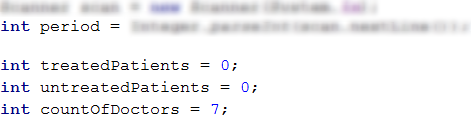The period which we have to make the calculations is read from the console and we store it in the variable period. We are going to need also some auxiliary variables: number of treated patients (treatedPatients), number of untreated patients (untreatedPatients), and the number of doctors (countOfDoctors), which by default is 7.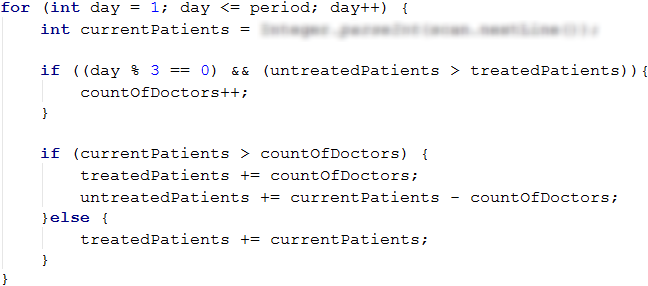Using a for loop, we iterate through all days in the given period (period). For each day, we read from the console the number of patients (currentPatients). Incrementing the number of doctors by the condition can be done every third day, BUT if only the number of the untreated patients is greater than the treated ones. For this purpose, we check if the day is the third one, using the arithmetical operator for division with the remainder (%): day % 3 == 0.

For example:

• If it’s the third one, the remainder from the division by 3 will be 0 (3 % 3 = 0) and the check day % 3 == 0 will return true.
• If it’s the second one, the remainder from the division by 3 will be 2 (2 % 3 = 2) and the check will return false.
• If it’s the fourth one, the remainder from the division will be 1 (4 % 3 = 1) and again the check will return false.

If the check day % 3 == 0 returns true, the program will check if the untreated patient’s count is greater than the treated ones: untreatedPatients > treatedPatients. If again the result is true, then we’ll increase the number of doctors (countOfDoctors).

After that, we check the number of patients for the day (currentPatients) whether it’s greater than the number of doctors (countOfDoctors). If the patient number is greater:

• We increase the value of the variable treatedPatients with the number of doctors (countOfDoctors).
• We increase the value of the variable untreatdPatients with the number of the rest of the patients, which we calculate, by subtracting the number of the doctors from all the patients (currentPatients - countOfDoctors).

If the patient count is less, we increase only the variable treatedPatients with the number of patients for the day (currentPatients).

Finally, we only need to print the number of treated and untreated patients.

### Problem: Division

There are n integer numbers given in the range of [1 … 1000]. From them, some percentage p1 is divisible without remainder by 2, percentage p2 is divisible by 3 without remainder, percentage p3 is divisible by 4 without remainder. Write a program that calculates the percentages of p1, p2 and p3, and prints them out. Example: We have n = 10 numbers: 680, 2, 600, 200, 800, 799, 199, 46, 128, 65. We get the following distribution and visualization:

Division without remainder by: Numbers Count Percent
2 680, 2, 600, 200, 800, 46, 128 7 p1 = (7 / 10) * 100 = 70.00%
3 600 1 p2 = (1 / 10) * 100 = 10.00%
4 680, 600, 200, 800, 128 5 p3 = (5 / 10) * 100 = 50.00%

### Input Data

On the first line of input is the integer number n (1 ≤ n ≤ 1000) – count of numbers. On the next n lines, we have one integer number in the range of [1 … 1000] – the numbers that have to be checked for division.

### Output Data

Print on the console 3 lines, each one containing a percentage between 0% and 100%, with accuracy up to two symbols after the decimal point, for example 25.00%, 66.67%, 57.14%.

• On the first line – the percentage of numbers that are divisible by 2.
• On the second line – the percentage of numbers that are divisible by 3.
• On the third line – the percentage of numbers that are divisible by 4.

### Sample Input and Output

Input Output Input Output Input Output
10
680
2
600
200
800
799
199
46
128
65
70.00%
10.00%
50.00%
3
3
6
9
33.33%
100.00%
0.00%
1
12
100.00%
100.00%
100.00%

### Hints and Guidelines

For the current problem and the next one, you’ll need to write the code by yourself, following the given guidelines.

The program that solves the current problem is analogous to the Histogram problem, which we reviewed earlier. Therefore, we can start with declaring the needed variables: Exemplary names for the variables can be n – count of the numbers (that we have to read from the console) and divisibleBy2, divisibleBy3, divisibleBy4 – auxiliary variables, containing the count of numbers in the corresponding group.

To read and assign every number in its corresponding group, we should iterate the for loop from 0 to n (count of numbers). Each iteration of the loop must read and allocate only one number. The difference here is that a number can end up in multiple groups simultaneously, that’s why we should create three separate if statements for each number – respectively whether it is divisible by 2, 3, and 4 (the if-else statement, in this case, won’t be useful because after finding a match it stops further checking of conditions) and increase the value of the variable that keeps the count of numbers for the corresponding group.

Finally, we have to print out the obtained results, by following the specified formatting.

### Problem: Logistics

It’s your responsibility for the logistics of various types of cargo. Depending on the weight of each load, a different vehicle type is needed, and the costs will vary per ton:

• Up to 3 tonsvan (200 USD per ton).
• Upward of 3 and up to 11 tonslorry (175 USD per ton).
• Over 11 tons – train (120 USD per ton).

It’s your problem to calculate, the average price per ton of transported cargo, and also what percentage of cargo is transported by the various vehicle types.

### Input Data

From the console, we must read a sequence of numbers, each on a separate line:

• First line: cargo count for transport – an integer number in the range of [1 … 1000].
• On each of the next lines, we input the weight of the current cargo – an integer number in the range of [1 … 1000].

### Output Data

Print out on the console 4 lines, as follows:

• Line #1 – an average price per ton of cargo (rounded up to the second symbol after the decimal point).
• Line #2 – a percentage of cargo, transported by van (between 0.00% and 100.00%, rounded up to the second symbol after the decimal point).
• Line #3 – a percentage of cargo, transported by lorry (between 0.00% and 100.00%).
• Line #4 – a percentage of cargo, transported by train (between 0.00% and 100.00%).

### Sample Input and Output

Input Output Explanations
4
1
5
16
3
143.80
16.00%
20.00%
64.00%
Two of the cargoes are transported by van 1 + 3, in total 4 tons.
One of the shipments is transported by lorry: 5 tons.
One of the shipments is transported by train: 16 tons.
The sum of all the cargo is: 1 + 5 + 16 + 3 = 25 tons.
Percentage cargo by van: 4/25*100 = 16.00%
Percentage cargo by lorry: 5/25*100 = 20.00%
Percentage cargo by train: 16/25*100 = 64.00%
Average price per ton of transported cargo: (4 * 200 + 5 * 175 + 16 * 120) / 25 = 143.80
Input Output Input Output
5
2
10
20
1
7
149.38
7.50%
42.50%
50.00%
4
53
7
56
999
120.35
0.00%
0.63%
99.37%

### Hints and Guidelines

First, we have to read the weight of each cargo and will sum up how many tons are transported by van, lorry, and train and we are going to calculate the total tons of transported cargo. We will calculate the prices for each type of transport determined by the total transported tonnage and the total price. In the end, we’ll calculate and print the total average price per ton and how much cargo is being transported by each transport type.

We declare the needed variables, for example: countOfLoads – the number of loads to be transported (we read them from the console), sumOfTons – the sum of the total weight of all cargo, vanTons, truckTons, trainTons – variables that keep the sum of the weight, transported by van, lorry, and train.

We are going to need a for loop from 0 to countOfLoads-1 to iterate through all the shipments. For each shipment, we read its weight (in tons) from the console and we save it to a variable, for example, tons. We add up to the tonnage sum of all cargos (sumOfTons) the weight of the current load (tons). After we’ve read the weight of the current cargo, we have to determine which transportation type we are going to use for it (van, lorry, or train). For this problem, we’re going to need if-else statements:

• If the value of the variable tons is less than 3, increase the value of vanTons by the value of tons:

vanTons += tons;

• Otherwise, if the value of tons is up to 11, we increase truckTons by tons.

• If tons are more than 11, we increase trainTons by tons.

Before we print out the output, we have to calculate the tonnage percentage of each transportation type and the average price per ton. For the average price, we are going to need one more auxiliary variable totalPrice, in which we are going to add up the total price of all transported cargo (by van, lorry, and train). For us to get the average price, we have to divide totalPrice by sumOfTons. It remains to calculate the percentages of the tonnage, transported by each transport type, and print out the results, adhering to the formatting specified in the description.